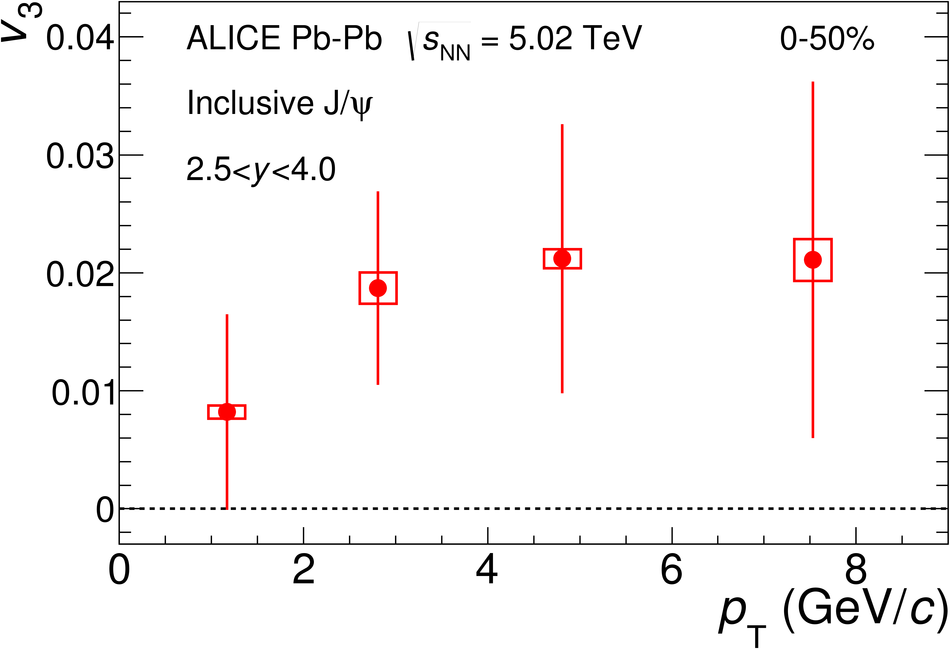# Figure 5

 The \jpsi\ $v_3$ coefficient as a function of $p_{\rm T}$ in the 0--50\ centrality interval in Pb--Pb collisions at $\sqrt{s_{\rm NN}}$ = 5.02 TeV. Statistical and systematic uncertainties are shown as bars and boxes, respectively.